# Monotone function

(diff) ← Older revision | Latest revision (diff) | Newer revision → (diff)

A function of one variable, defined on a subset of the real numbers, whose increment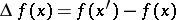, for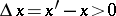, does not change sign, that is, is either always negative or always positive. Ifis strictly greater (less) than zero when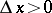, then the function is called strictly monotone (see Increasing function; Decreasing function). The various types of monotone functions are represented in the following table.'

<tbody> </tbody>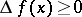Increasing (non-decreasing)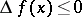Decreasing (non-increasing)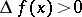Strictly increasing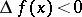Strictly decreasingIf at each point of an intervalhas a derivative that does not change sign (respectively, is of constant sign), thenis monotone (strictly monotone) on this interval.

The idea of a monotone function can be generalized to functions of various classes. For example, a functiondefined onis called monotone if the condition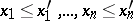implies that everywhere either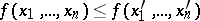or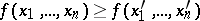everywhere. A monotone function in the algebra of logic is defined similarly.

A monotone function of many variables, increasing or decreasing at some point, is defined as follows. Letbe defined on the-dimensional closed cube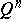, let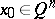and let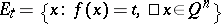be a level set of. The functionis called increasing (respectively, decreasing) atif for anyand any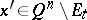not separated inby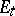from, the relation(respectively,) holds, and for any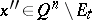that is separated inbyfrom, the relation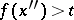(respectively,) holds. A function that is increasing or decreasing at some point is called monotone at that point.

How to Cite This Entry:
Monotone function. Encyclopedia of Mathematics. URL: http://encyclopediaofmath.org/index.php?title=Monotone_function&oldid=18679
This article was adapted from an original article by L.D. Kudryavtsev (originator), which appeared in Encyclopedia of Mathematics - ISBN 1402006098. See original article